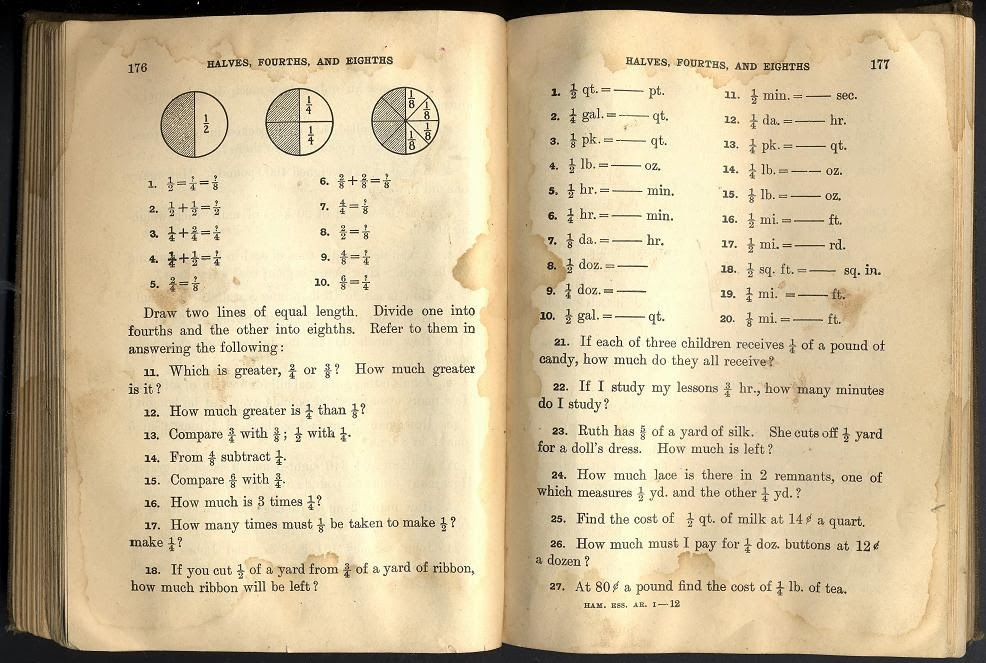@Reks: Educational iOS Applications

## ABC Printable WorksheetsLearn letters of the English alphabet and practice writing letters with these printable worksheets.

## 4th-Grade Printable Math QuizSheetsThis page offers a number of math worksheets for Grade 4 following Math Essentials math textbook and common core standards. The worksheets are organized in four different lessons (Units). The Math topics in the first lesson (Unit) are as follows: – Calculate area and perimeter of triangle, square, rectangle, parallelogram, or trapezoid – Order of […]